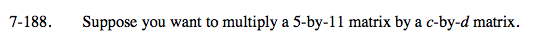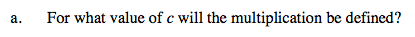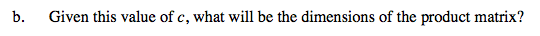Home > A2C > Chapter 7 > Lesson 7.3.3 > Problem7-188

7-188.
1. Suppose you want to multiply a 5-by-11 matrix by a c-by-d matrix. Homework Help ✎

1. For what value of c will the multiplication be defined?

2. Given this value of c, what will be the dimensions of the product matrix?The multiplication will only be defined if c = 11.The product matrix will be a 5-by-d matrix.